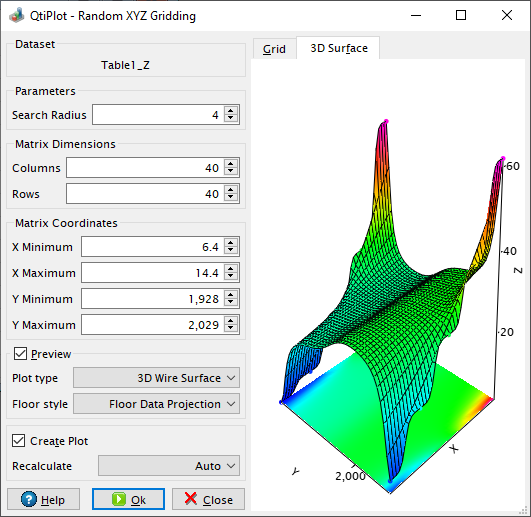# Random XYZ Gridding Dialog

This dialog is opened when you select the Convert to Matrix -> Random XYZ... command from the Convert to Matrix menu. It allows to customize the process of generating a new matrix from a collection of randomly distributed XYZ data samples, using Shepard's method.

This interpolation method, also called the Inverse distance weighting method, is often used to derive estimates of the surface height at the vertices of a regular grid from irregularly spaced samples. Consider N data samples, that is, we have N triples (xi, yi, zi). We want to estimate the height z given a position on the plane (x, y). The general form for estimating z is given by the following formula, where p determines the relative importance of distant samples (see the article Nearest neighbour weighted interpolation by Paul Bourke for more explanations):

Figure 5-156. The Shepard's interpolation method.The Search Radius input box in the dialog corresponds to the parameter p from the formula above. The initial dimensions of the output matrix are automatically set by QtiPlot to be the double of the number of sample data points, but of course you can customize them via the Columns and Rows input boxes from the Matrix Dimensions group box. The same remark applies to the controls in the Matrix Coordinates group box.

By checking/unchecking the Preview box it is possible to enable/disable the display of the input samples and of the interpolated 3D surface. The color map used to draw the input data points as well as the color of the surface line mesh and its smootheness can be customized via the 3D Plots tab from the Preferences dialog.

Figure 5-157. The Random XYZ Gridding dialog box.